Distributed Programming
Lecture 02 - Processes, Threads and
Synchronization
Edirlei Soares de LimaPrograms and Processes
What is a computer program?
Is a sequence of instructions for performing specific tasks or solve
specific problems;
Static entity (an .exe file on Windows);
What is a process?
Is an instance of a computer program that is being executed (more
than one process can run the code of the same program);
Dynamic entity that changes its internal state while running the
program code;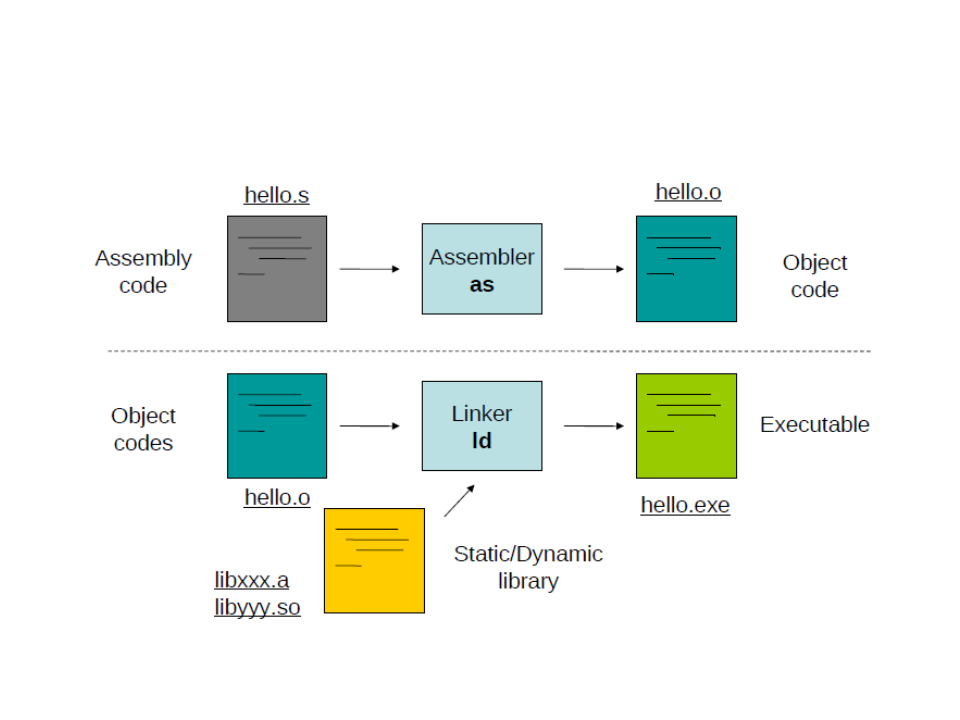Compilation Process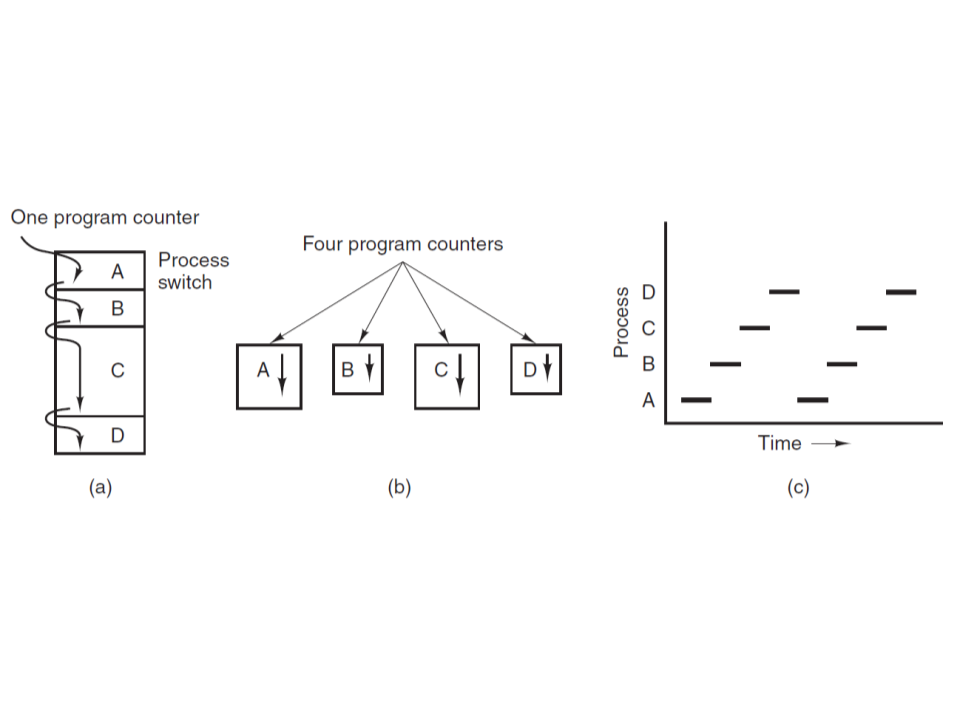Process Model
(
(
(
a) Multiprogramming four programs (the CPU switches back and forth
from process to process).
b) Conceptual model of four independent, sequential processes (its
easier to think that the processes are running in (pseudo) parallel).
c) Only one program is active at once.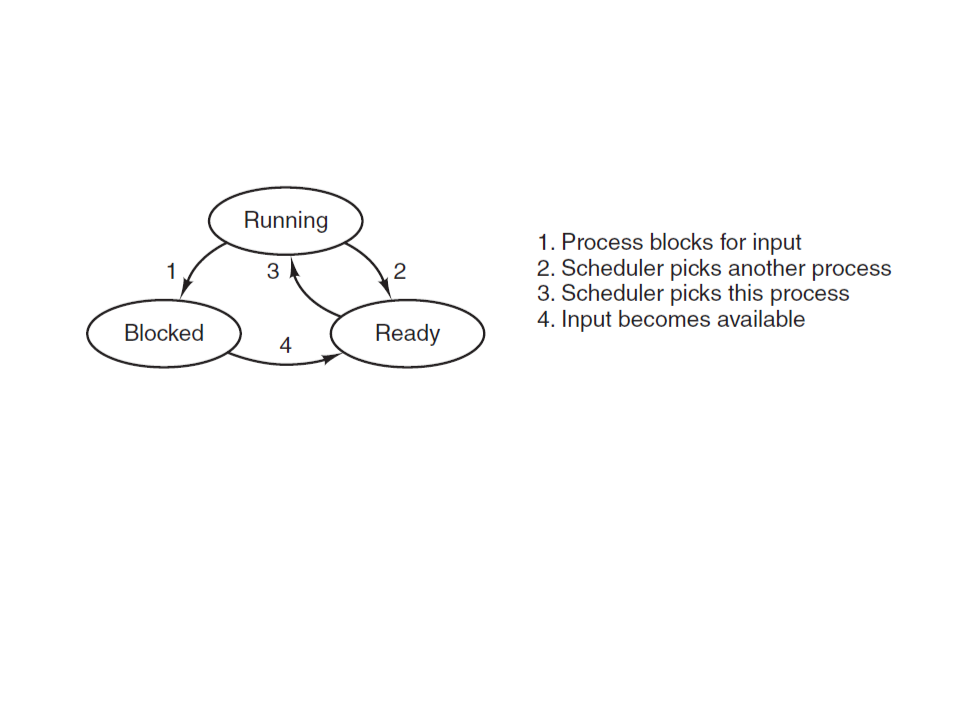Process States
Running (actually using the CPU at that instant).
Ready (runnable; temporarily stopped to let another process run).
Blocked (unable to run until some external event happens).control.
However, in many situations it is desirable to have multiple threads of
control in the same address space running in parallel.
Multiple threads can exist within one process, executing
concurrently and sharing resources.
Threads are faster to create and destroy than processes (10 to
1
00 times faster) and take advantage of multiple CPU cores.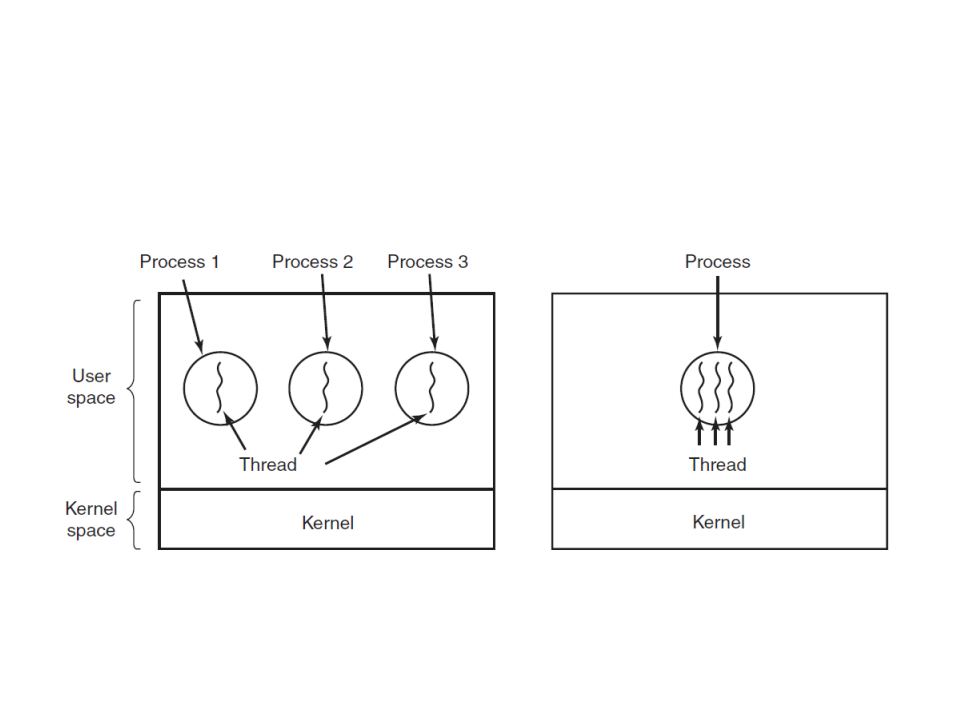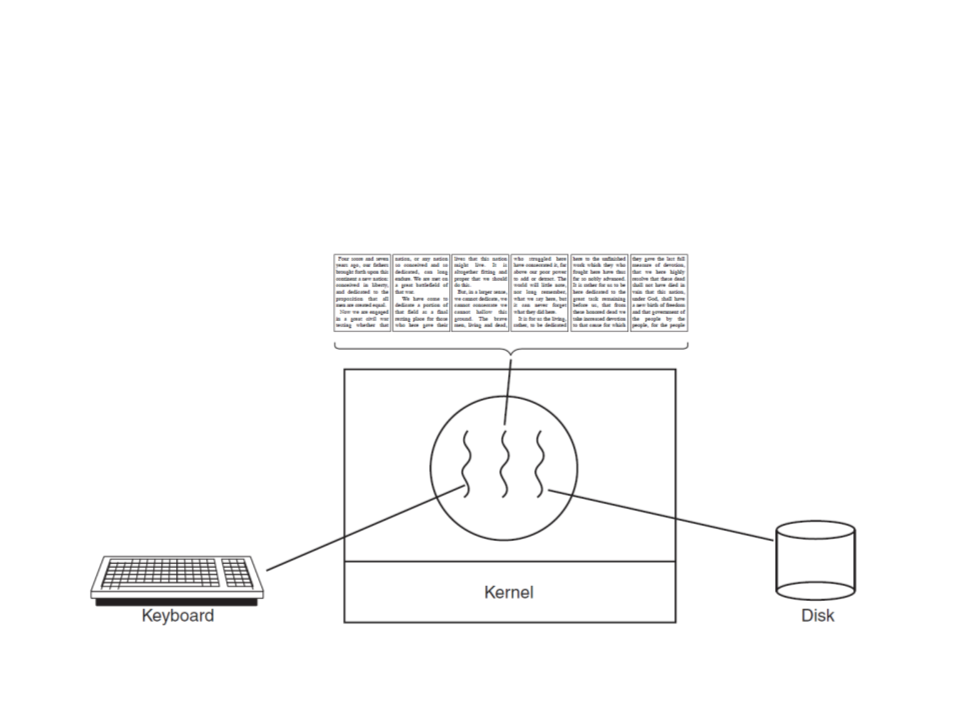Word Processor: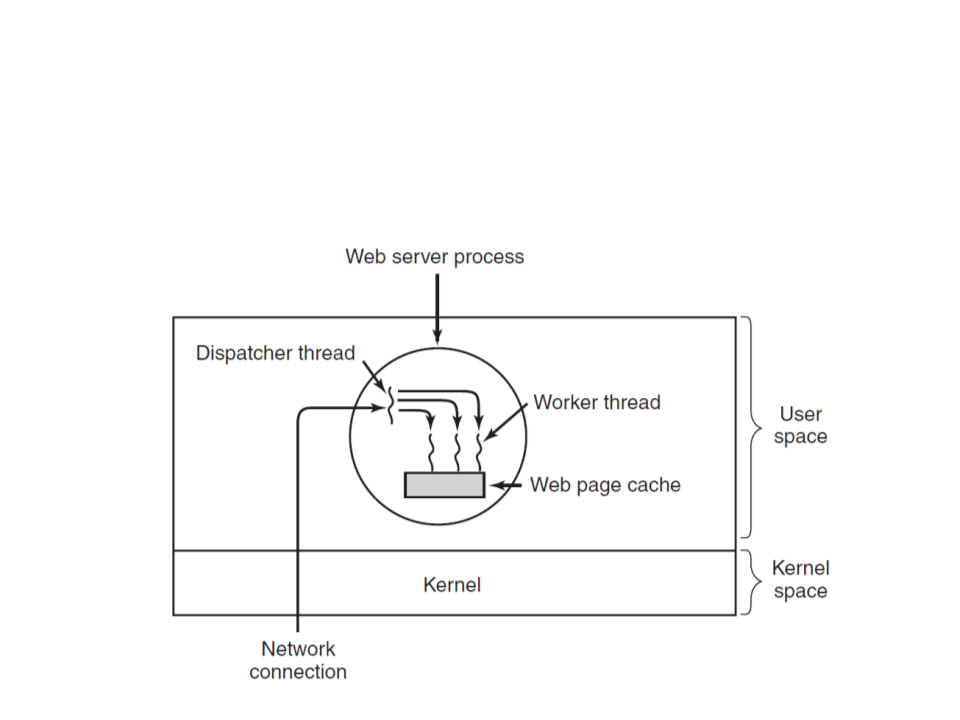Web Server:#
#
include <iostream>
{
std::cout << "Hello Thread World!" << std::endl;
}
int main()
{
std::cout << "End Program!" << std::endl;
return 0;
}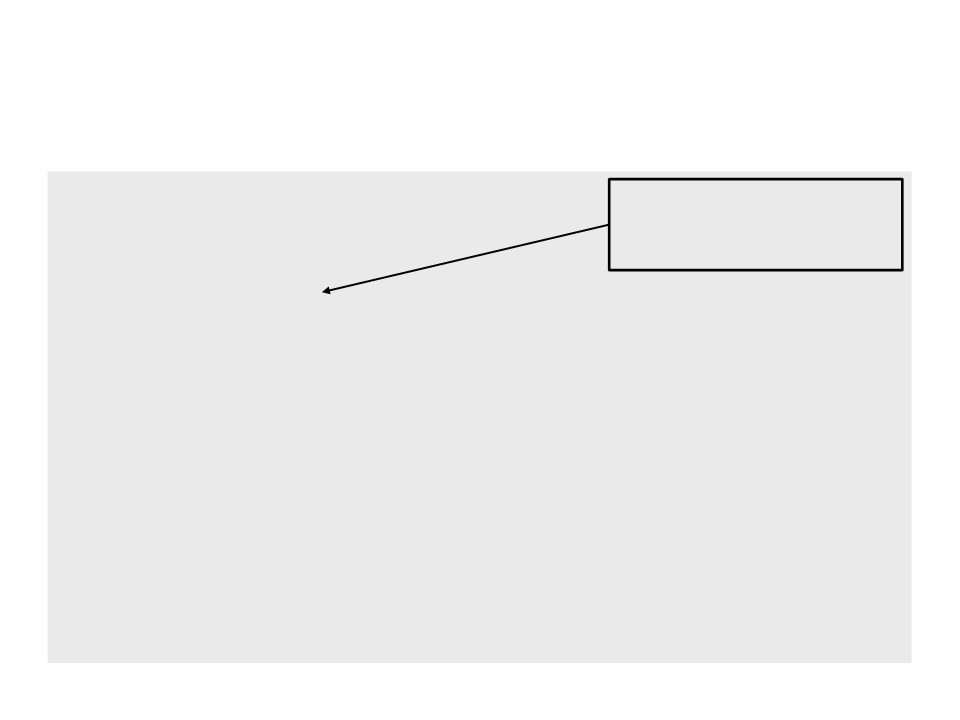#
#
#
include <iostream>
In this way, we don’t need
to indicate the namespace
include <vector>
all the time.
using namespace std;
{
for (int i = 1; i < 10; i++)
{
cout << "Thread " << threadID << " - Lap " << i << endl;
}
cout << "Thread " << threadID << " Arrived! " << endl;
}
......
int main()
{
srand(time(NULL));
for (int i = 0; i < 5; i++)
{
}
for (int i = 0; i < 5; i++)
{
}
cout << "End Program!" << endl;
return 0;
}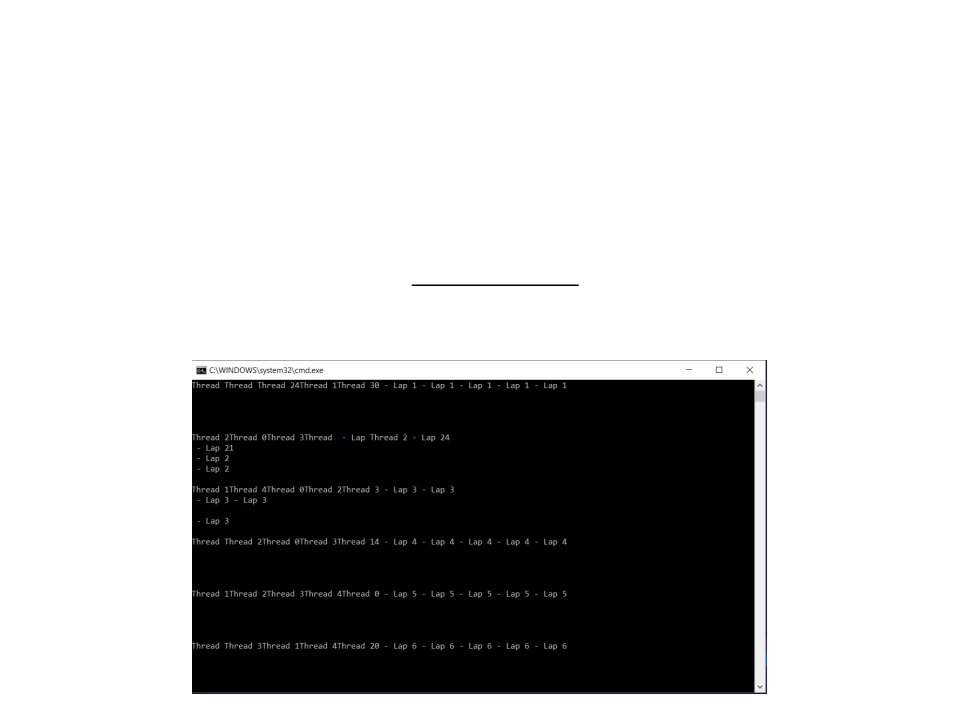What is wrong with the results?
Threads are printing their messages at the same time!
This problem is know as “Race Condition”. It occurs when two or more
processes are reading or writing some shared data (in this case, the
console).Race Conditions
How do we avoid race conditions?
The key to preventing problems like this is to find some way to prohibit
more than one process from reading and writing the shared data at
the same time.
We need a way of making sure that if one process is using a
shared variable or file, the other processes will be excluded
from doing the same thing.
The part of the program where the shared data is accessed is
called the critical region or critical section.Race Conditions
Naive solution: Lock Variables
Consider having a single, shared (lock) variable, initially 0. When a
process wants to enter its critical region, it first tests the lock. If the
lock is 0, the process sets it to 1 and enters the critical region. If the
lock is already 1, the process just waits until it becomes 0.
int lock = 0;
for (int i = 1; i < 10; i++){
bool lap = false;
while (lap == false){
if (lock == 0){
lock = 1;
cout << "Thread " << threadID << " - Lap " << i << endl;
lock = 0;
lap = true;
}
}
...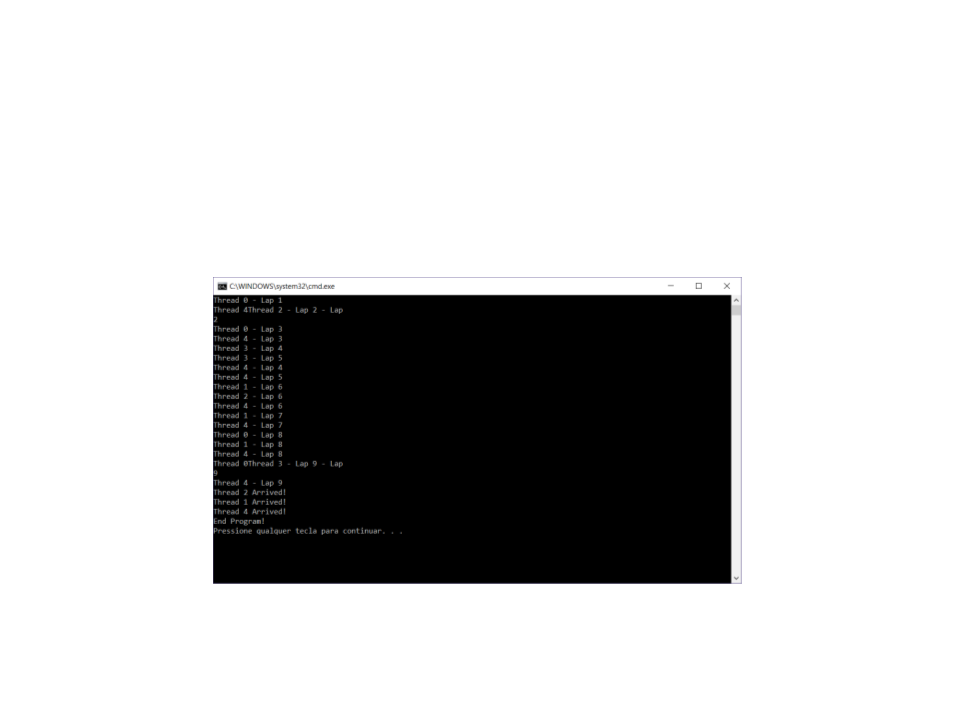Race Conditions Naive Solution
It solves the problem?
It reduces the chances, but don’t solve completely the problem…
Why?
The race condition now occurs if the second process modifies the lock
just after the first process has finished the lock check.Race Conditions Mutual Exclusion
Solution: Mutex
.
#
.
..
include <mutex>
..
mutex mymutex;
for (int i = 1; i < 10; i++){
mymutex.lock();
cout << "Thread " << threadID << " - Lap " << i << endl;
mymutex.unlock();
}
mymutex.lock();
cout << "Thread " << threadID << " Arrived! " << endl;
mymutex.unlock();
}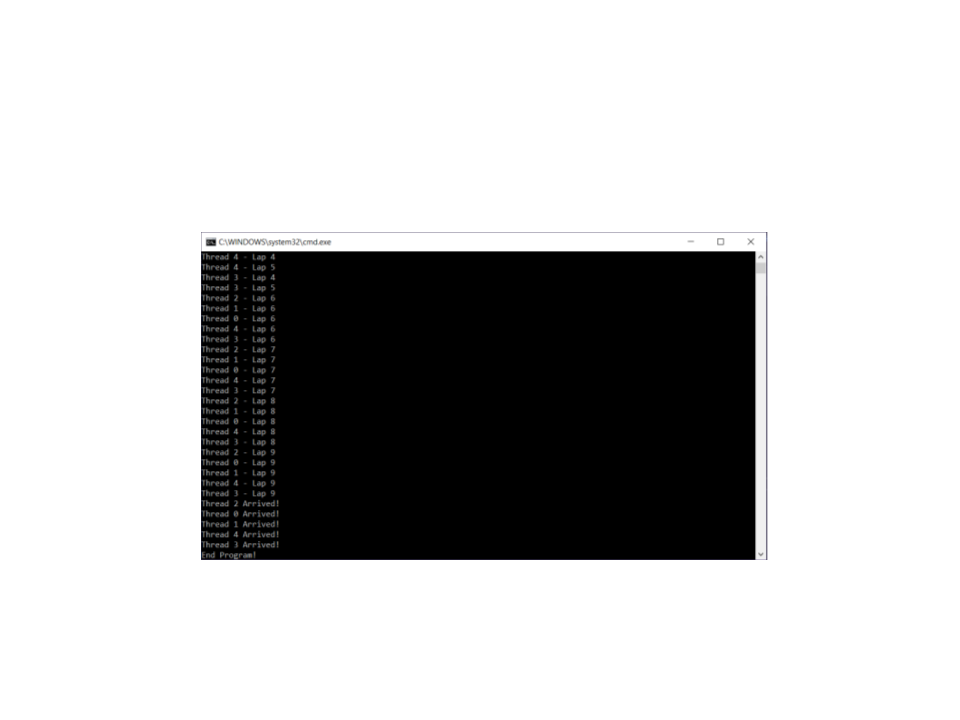Race Conditions Mutual Exclusion
Mutex solves the problem:
What happens to the other threads while one is inside the critical region?
Busy-waiting! (wasting a lot of CPU time).Exercise 1
1
) Implement a program in C++ to sum the values of each row
of a 5x5 matrix and display the results on screen.
must be responsible for computing the sum of a single row.
The matrix can be statically declared. Example of 3x3 matrix:
int mymatrix = {{10,20,30},{40,50,60},{70,80,90}};Exercise 2
2
) Implement a program in C++ to multiply the values of an
array (with 1000 int values) by a scalar and display the
resulting array on screen.
The multiplication process must be done in parallel using 10 thread,
where each thread must be responsible for multiplying 100 elements
of the array by the scalar.
The results of the multiplications must be stored in another array.Supposing that x * x is a very costly operation (it's not, but use
your imagination). Can we parallelize the calculation of the
sum of squares up to a certain number?int cont = 0;
void square(int x)
{
cont += x * x;
}
int main()
{
for (int i = 1; i <= 20; i++){
}
for (int i = 1; i <= 20; i++){
}
cout << "Count = " << cont << endl;
return 0;
}If you run the program, chances are it outputs 2870 (which is
the correct answer). But after few executions, you probably
will see some wrong results:
If you never see a wrong result, your computer is running the thread
code too fast. You can “solve” this by adding a sleep time to the thread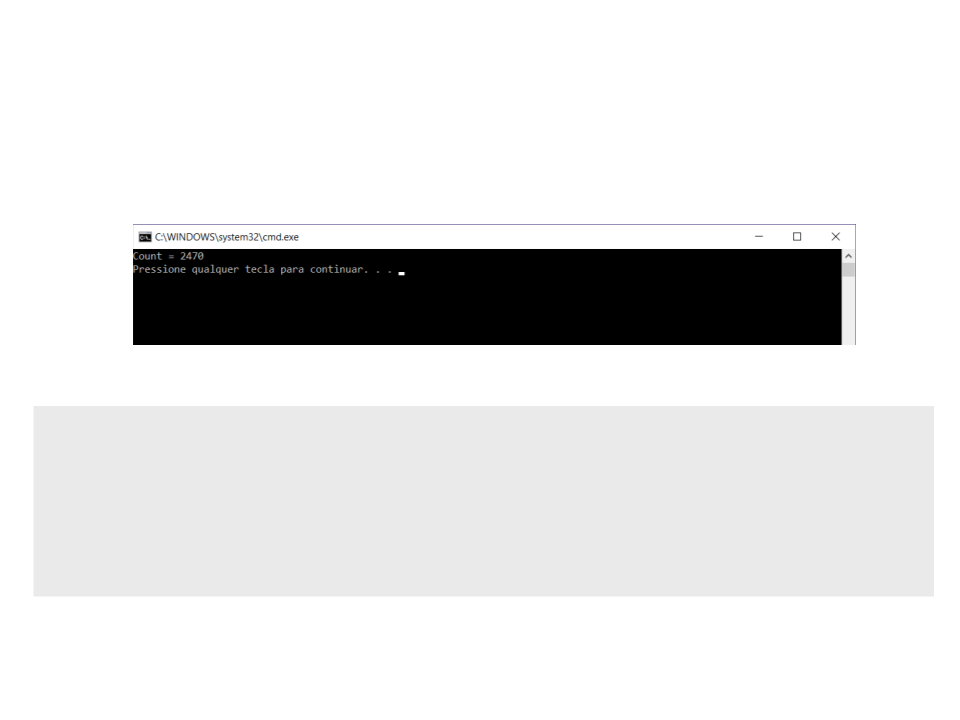This is caused by another race condition. Why it happens?
Example:
int temp1 = cont;
// temp1 = 0
// temp2 = 0
// temp2 = 4
// temp1 = 1
// cont = 1
// cont = 4
int temp2 = cont;
temp2 += 2 * 2;
temp1 += 1 * 1;
cont = temp1;
cont = temp2;
We end up with cont as 4, instead of the correct 5.We can also solve this problem using mutex:
.
#
.
..
include <mutex>
..
mutex mymutex;
void square(int x)
{
mymutex.lock();
cont += x * x;
mymutex.unlock();
}C++ offers an even nicer abstraction to this problem: atomic
contained value from different threads cannot cause race conditions.
.
#
.
..
include <atomic>
..
atomic<int> cont(0);
void square(int x)
{
cont += x * x;
}C++ provides an even higher level of abstraction that avoids
Simple example:
#
#
include <iostream>
The async construct uses an object pair called a
include <future>
promise and a future: the future is linked to the
promise and can at any time try to retrieve the
value (get()). If the promise hasn't been fulfilled
yet, it will simply wait until the value is ready.
using namespace std;
int square(int x){
return x * x;
}
int main(){
future<int> mytask = async(launch::async, square, 10);
cout << "The thread returned " << value << endl;
return 0;
}We can solve the sum of squares problem using tasks:
#include <iostream>
#include <vector>
#include <future>
using namespace std;
int square(int x)
{
return x * x;
}
...int main()
{
for (int i = 1; i <= 20; i++)
{
}
int cont = 0;
for (int i = 1; i <= 20; i++)
{
}
cout << "Count = " << cont << endl;
return 0;
}Condition Variables
Sometimes, its useful to be able to have one thread wait for
another thread to finish processing something before doing
To do that, we need a way to send signals between threads.
Naive solution:
Use a global boolean variable that is set to true when we want to send
the signal. The other thread would then run a loop that checks if
variable is true and stops looping when that happens.
Problem: the receiving thread will be running a loop at full speed
(wasting a lot of CPU time).Condition Variables: Naive Solution
#
#
include <iostream>
using namespace std;
int producedData = 0;
bool notified = false;
void Assigner()
{
producedData = 42;
notified = true;
}
void Reporter()
{
while (!notified) { /*do nothing */ }
cout << "Data: " << producedData << endl;
}Condition Variables: Naive Solution
int main()
{
assigner.join();
reporter.join();
return 0;
}
It works, but the while loop in the reporter thread will be running a
loop at full speed (wasting a lot of CPU time).
Better solution: C++ condition variablesC++ Condition Variables
#
#
#
#
include <iostream>
include <condition_variable>
include <mutex>
using namespace std;
condition_variable mycondvar;
mutex mymutex;
int producedData = 0;
void Assigner()
{
producedData = 42;
mycondvar.notify_one();
}
...C++ Condition Variables
...
void Reporter()
{
unique_lock<mutex> lock(mymutex);
mycondvar.wait(lock);
cout << "Data: " << producedData << endl;
}
int main()
{
assigner.join();
reporter.join();
return 0;
}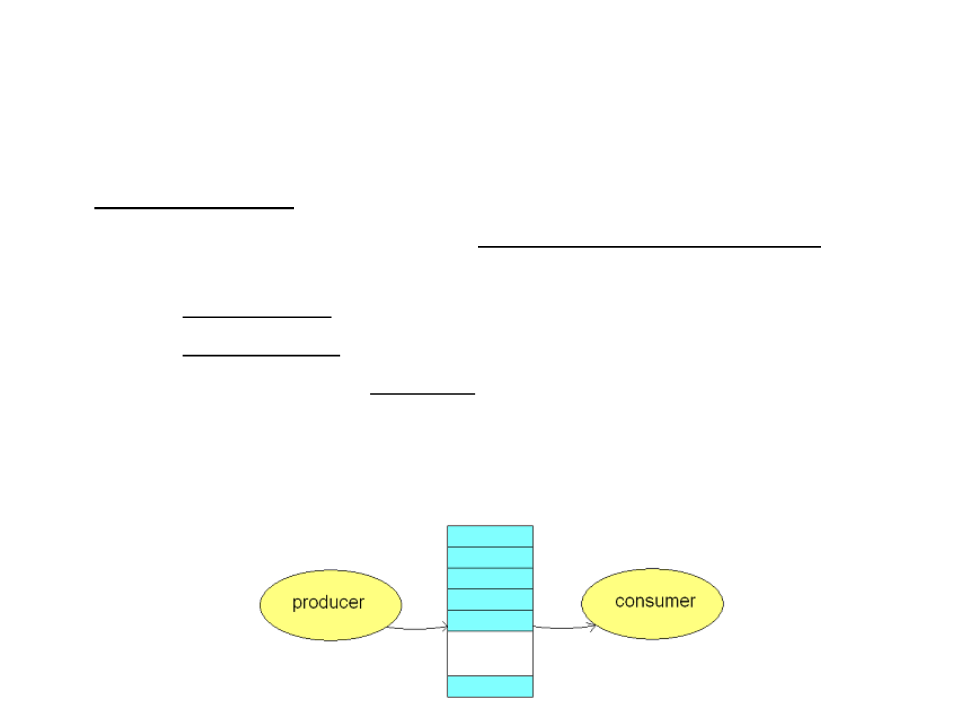Producer-Consumer Problem
Game Scenario:
Two independent NPCs share a common container of resources (e.g.
food, ammo, etc.) with a limited capacity.
The producer task is to gather resources and put it in the container.
The consumer task it to remove resources from the container.
Both can only handle one piece of resource at a time.
The problem is to ensure that the producer never tries to put
resources in when the container is full and the consumer never tries to
remove resources from the container when it is empty.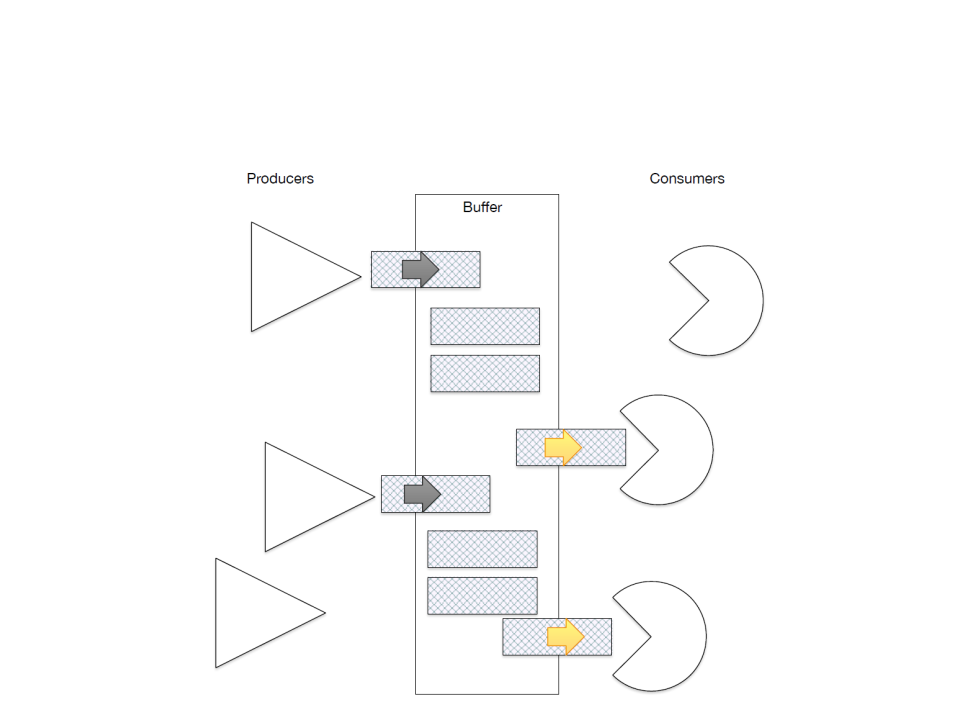Producer-Consumer ProblemProducer-Consumer Problem
Examples of Applications:
Factory scenario:
Robots hang parts coming from a production line on a rack.
Robot operators pick one part at a time from the rack to assemble the
final product.
Web service:
The web service receives http requests for data, places the request into an
internal queue.
Worker thread pulls the work item from the queue and performs the
work.PRODUCER:
CONSUMER:
1
2
3
4
5
. if buffer is full
. go to sleep
1. if buffer is empty
2. go to sleep
2
1
3
. put new item in buffer 3. remove item from buffer
. if buffer has 1 item
. wake consumer
4. if buffer has free slots
5. wake producerProducer-Consumer: Base Code
#include <iostream>
#include <queue>
using namespace std;
queue<int> resources;
int totalConsumed = 0;
int totalProduced = 0;
bool producionDone = false;
void Producer()
{
for (int i = 0; i < 500; i++)
{
resources.push(i);
totalProduced++;
}
producionDone = true;
}Producer-Consumer: Base Code
void Consumer(){
while (!producionDone){
while (!resources.empty()){
resources.pop();
totalConsumed++;
}
}
}
int main(){
producer.join();
consumer.join();
cout << "Total Produced: " << totalProduced << endl;
cout << "Total Consumed: " << totalConsumed << endl;
cout << "Total Container: " << resources.size() << endl;
return 0;
}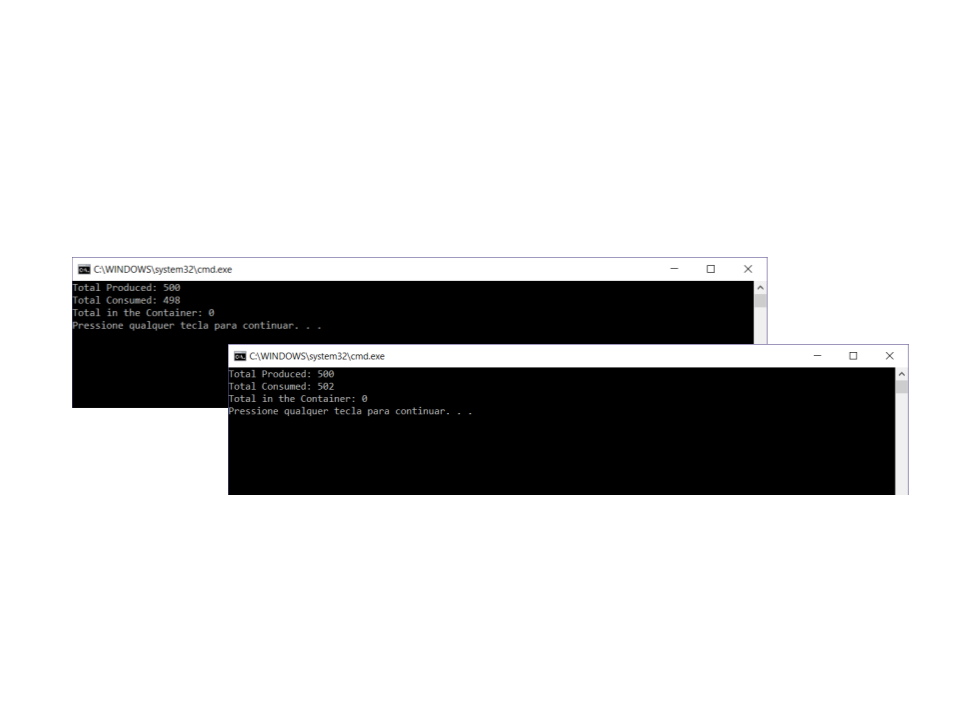Producer-Consumer Problem
Problem:
What is wrong with the code?Exercise 3
3
) Correct the problems in the implementation of Producer-
Consumer using condition variables and mutexes.
Ensure that the producer never tries to put resources in when the
container is full (define a constant to represent the capacity of the
container).
A simple mutex can solve the problem, but is not the best solution
(busy waiting problem). Use condition variables to synchronize the